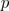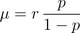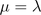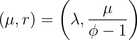Want to share your content on R-bloggers? click here if you have a blog, or here if you don't.

Here
and there, I mentioned two codes to generated quasiPoisson random
variables. And in both of them, the negative binomial approximation
seems to be wrong. Recall that the negative binomial distribution iswhereand in R, a negative binomial distribution can be parametrized using
two parameters, out of the following ones

• the size• the probability• the meanRecall also that the variance for a negative binomial distribution
should beHere, we consider a distribution such thatand. In the previous posts, I usedi.e.

`rqpois = function(n, lambda, phi) {mu = lambdak = mu/(phi * mu - 1)r1 = rnbinom(n, mu = mu, size = k)r2 = rnbinom(n, size=phi*mu/(phi-1),prob=1/phi)k = mu/phi/(1-1/phi)r3 = rnbinom(n, mu = mu, size = k)r4 = rnbinom(n, size=mu/phi/(1-1/phi),prob=1/phi)r = cbind(r1,r2,r3,r4)return(r)}`

but as we can see below, none of those two functions work,

`> N=rqpois(1000000,2,4)> mean(N[,1]) 2.001992> mean(N[,2]) 8.000033> var(N[,1])/ mean(N[,1]) 7.97444> var(N[,2])/ mean(N[,2]) 4.002022`

with the first one, the expected value is correct, while it is the
overdispersion parameter for the second. Now, if we do the maths
correctly, it comeswhich should now work,

`> mean(N[,3]) 2.001667> mean(N[,4]) 2.002776> var(N[,3])/ mean(N[,3]) 3.999318> var(N[,4])/ mean(N[,4]) 4.009647`

So, finally it is better when we do the maths well.